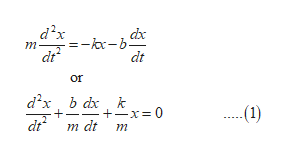# Mechanical Vibrations (differential equations)A mass weighing 4 pounds is attached to a sping whose constant is 2lb/ft. The medium offers a damping force that is numerically equal to the instantaneous velocity. The mass is initially released from a point one foot above the equilibrium position with a downward velocity of 8ft/s. Determine the time at which the mass passes through the equilibrium position.

Question
18 views

Mechanical Vibrations (differential equations)

A mass weighing 4 pounds is attached to a sping whose constant is 2lb/ft. The medium offers a damping force that is numerically equal to the instantaneous velocity. The mass is initially released from a point one foot above the equilibrium position with a downward velocity of 8ft/s. Determine the time at which the mass passes through the equilibrium position.

check_circle

Step 1

Let m be the mass attached, k be the spring constant and let b be a positive damping constant.

Then, by Newton’s second law for the system is:help_outlineImage Transcriptionclosed2x dxc m -b- dt dt or а?x, b dx, k +x0 m dt m (1) dt fullscreen
Step 2

Where x(t) is the displacement from the equilibrium position.

Now, determine the equation of motion.

Step 3

Now, put m=1/8 slugs, k=2 lb/ft and b=1 into t...

### Want to see the full answer?

See Solution

#### Want to see this answer and more?

Solutions are written by subject experts who are available 24/7. Questions are typically answered within 1 hour.*

See Solution
*Response times may vary by subject and question.
Tagged in

### Math